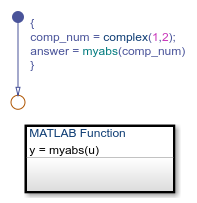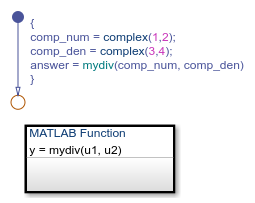## Best Practices for Using Complex Data in C Charts

Complex data is data whose value is a complex number. For example, in a Stateflow® chart in Simulink® model, an input signal with the value `3 + 5i` is complex. See Complex Data in Stateflow Charts.

When you use complex data in Stateflow charts that use C as the action language, follow these best practices.

### Perform Math Function Operations with a MATLAB Function

Math functions such as `sin`, `cos`, `min`, `max`, and `abs` do not work with complex data in C charts. However, you can use a MATLAB® function in your chart to perform math function operations on complex data.

#### A Simple Example

In the following chart, a MATLAB function calculates the absolute value of a complex number:The value of `comp_num` is `1+2i`. Calculating the absolute value gives an answer of `2.2361`.

#### How to Calculate Absolute Value

Suppose that you want to find the absolute value of a complex number. Follow these steps:

`y = myabs(u)`
2. Double-click the function box to open the editor.

3. In the editor, enter the code below:

```function y = myabs(u) %#codegen y = abs(u);```

The function `myabs` takes a complex input `u` and returns the absolute value as an output `y`.

4. Configure the input argument `u` to accept complex values.

1. Open the Model Explorer.

2. In the Model Hierarchy pane of the Model Explorer, navigate to the MATLAB function `myabs`.

3. In the Contents pane of the Model Explorer, right-click the input argument `u` and select Properties from the context menu.

4. In the Data properties dialog box, select `On` in the Complexity field and click .

You cannot pass real values to function inputs of complex type. For details, see Rules for Using Complex Data in C Charts.

### Perform Complex Division with a MATLAB Function

Division with complex operands is not available as a binary or assignment operation in C charts. However, you can use a MATLAB function in your chart to perform division on complex data.

#### A Simple Example

In the following chart, a MATLAB function performs division on two complex operands:The values of `comp_num` and `comp_den` are `1+2i` and `3+4i`, respectively. Dividing these values gives an answer of `0.44+0.08i`.

#### How to Perform Complex Division

To divide two complex numbers:

1. Add a MATLAB function to your chart with this function signature:

`y = mydiv(u1, u2)`
2. Double-click the function box to open the editor.

3. In the editor, enter the code below:

```function y = mydiv(u1, u2) %#codegen y = u1 / u2;```

The function `mydiv` takes two complex inputs, `u1` and `u2`, and returns the complex quotient of the two numbers as an output `y`.

4. Configure the input and output arguments to accept complex values.

1. Open the Model Explorer.

2. In the Model Hierarchy pane of the Model Explorer, navigate to the MATLAB function `mydiv`.

3. For each input and output argument, follow these steps:

1. In the Contents pane of the Model Explorer, right-click the argument and select Properties from the context menu.

2. In the Data properties dialog box, select `On` in the Complexity field and click .

You cannot pass real values to function inputs of complex type. For details, see Rules for Using Complex Data in C Charts.

### Rules for Using Complex Data in C Charts

Complex data is data whose value is a complex number. For example, in a Stateflow chart in Simulink model, an input signal with the value `3 + 5i` is complex. See Complex Data in Stateflow Charts.

These rules apply when you use complex data in Stateflow charts that use C as the action language.